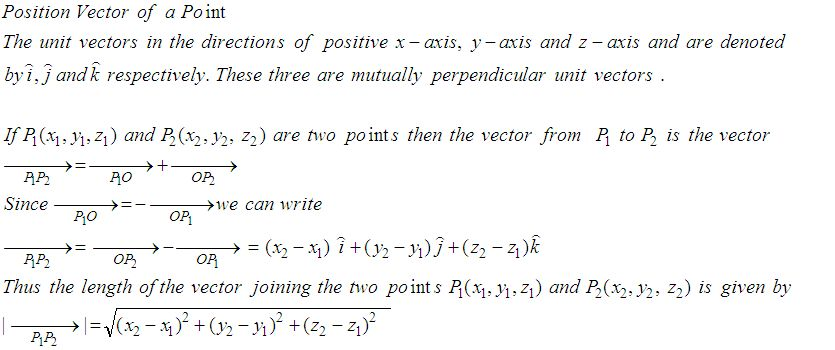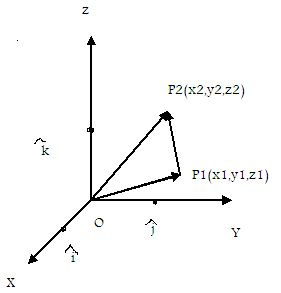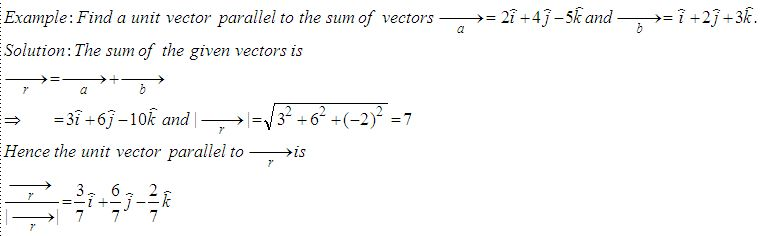Email us to get an instant 20% discount on highly effective K-12 Math & English kwizNET Programs!

Online Quiz (WorksheetABCD)

Questions Per Quiz = 2 4 6 8 10

High School Mathematics - 25.9 Position Vector of a PointDirections: Answer the following.
 Q 1: Find a unit vector parallel to the sum of vectors a = 2i+4j-5k, b = i+2j+3k3/7i + 6/7j - 2/7 k2-i88i+4j-3k Q 2: If P = 2,4,7 and P2 = (-4, -1, 5) then find vector P1P2.-6i-5j-2k-6i+5j6i+5j+3k Q 3: Find the condition that the vectors a = ki + 3j and b = 4i+kj, (k not equal to 0) are parallel.k2 = 12k = 0k = 6 Q 4: Find the vector with initial point P(6,-2) and terminal point Q(4,-8).6i-9j-2i+10j8i+2j Question 5: This question is available to subscribers only! Question 6: This question is available to subscribers only!